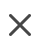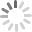# Glassware

Sort By |
1 - 23 of 23 Results
sku
common name
form
price
size
[ 111 ]
16oz
\$1.45
Each
Quantity:
[ 825 ]
8oz
\$1.35
OUT OF STOCK (ETA 5/25/2020)
Out of stock
[ 589 ]
4oz
\$1.05
Each
Quantity:
[ 818 ]
2oz
\$1.00
Each
Quantity:
[ 65 ]
1oz
\$0.90
Each
Quantity:
[ 715 ]
1/2oz
\$0.70
Each
Quantity:
[ 1702 ]
500cc
\$2.80
Each
Quantity:
[ 1566 ]
250cc
\$1.60
Each
Quantity:
[ 1701 ]
150cc
\$1.50
Each
Quantity:
[ 1705 ]
60cc
\$1.20
Each
Quantity:
[ 666 ]
1oz
\$1.40
Each
Quantity:
[ 207 ]
1 gallon
\$6.50
Each
Quantity:
[ 1081 ]
1/2 gallon
\$5.50
Each
Quantity:
[ 276 ]
2oz
\$1.30
Each
Quantity:
[ 862 ]
1oz
\$1.20
Each
Quantity:
[ 166 ]
8oz
\$1.65
Each
Quantity:
[ 1258 ]
4oz
\$1.20
Each
Quantity:
[ 1280 ]
1/4oz
\$0.90
Each
Quantity:
[ 576 ]
1/5 drams
\$0.20
Each
Quantity:
[ 1874 ]
2oz
\$0.70
Each
Quantity:
[ 1333 ]
1oz
\$0.60
Each
Quantity:
[ 1873 ]
1/2oz
\$0.55
Each
Quantity:
[ 38 ]
2oz
\$1.20
Each
Quantity:
1 - 23 of 23 Results